# Ex.3.4 Q4 Understanding Quadrilaterals Solution - NCERT Maths Class 8

Go back to  'Ex.3.4'

## Question

(i) bisect each other

(ii) are perpendicular bisectors of each other

(iii) are equal

Video Solution
Ex 3.4 | Question 4

## Text Solution

Steps:

(i) bisect each other

Parallelogram: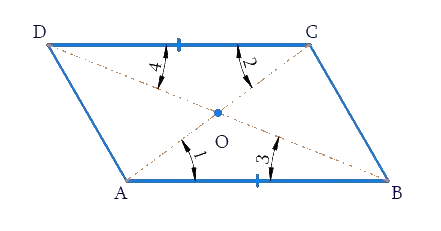Rhombus: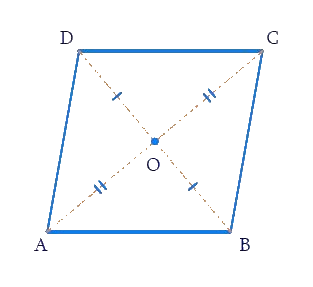Rectangle: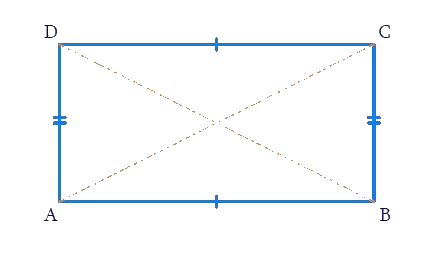Square: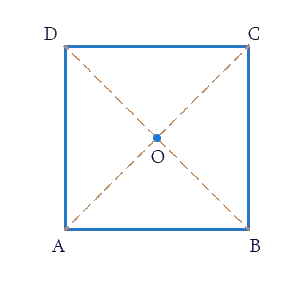a) Parallelogram

b) Rhombus

c) Rectangle

d) Square

The diagonals of a parallelogram, rhombus, rectangle and square are perpendicular bisectors of each other.

ii) Are perpendicular bisectors of each other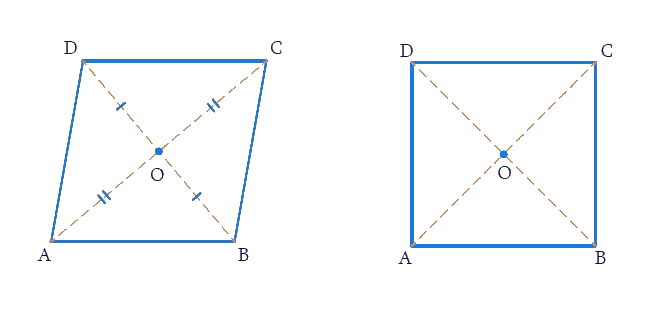a) Rhombus

b) Square

The diagonals of a square and rhombus are perpendicular bisectors of each other.

(iii) are equal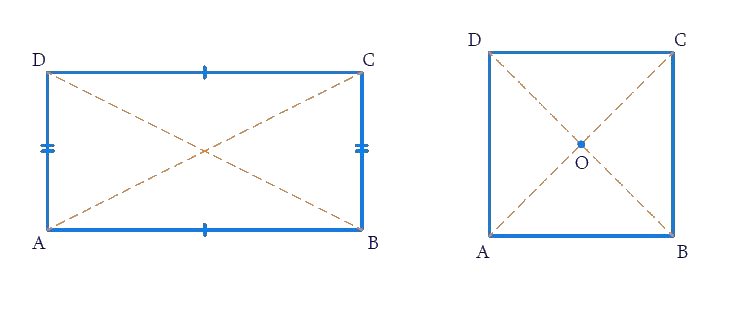a) Rectangle

b) Square

The diagonals of a rectangle and square are equal.

Learn from the best math teachers and top your exams

• Live one on one classroom and doubt clearing
• Practice worksheets in and after class for conceptual clarity
• Personalized curriculum to keep up with school